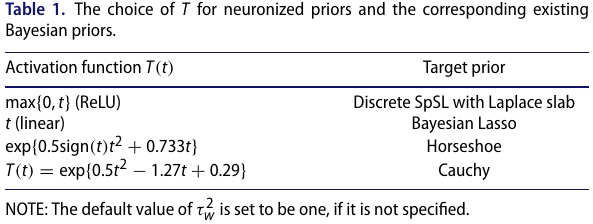# Neuronized Priors for Bayesian Sparse Linear Regression

##### Posted on Jan 16, 2022

Practically, the routine use of Bayesian variable selection methods has not caught up with the non-Bayesian counterparts such as Lasso, likely due to difficulties in both computations and flexibilities of prior choices.

The paper propose the neuronized priors to unify and extend some popular shrinkage priors, such as Laplace, Cauchy, horseshoe, and spike-and-slab priors.

A neuronized prior can be written as the product of a Gaussian weight variable and a scale variable transformed from Gaussian via an activation function.

Compared with classic spike-and-slab priors, the neuronized priors achieve the same explicit variable selection without employing any latent indicator variables.

Consider standard linear regression model,

$y=X\theta+\epsilon$

To model the sparsity of $\theta$ when $p$ is large, a popular choice is the one-group continuous shrinkage prior, which can be represented as a hierarchical scale-mixture of Gaussian distributions.

$\theta_j\mid \nu_w^2,\tau_j^2 \sim N(0, \nu_w^2\tau_j^2)\\ \tau_j^2\sim \pi_\tau, \nu_w\sim \pi_g$

where

• the local shrinkage parameter $\tau_j^2$ governs the shrinkage level of an individual parameter
• the global shrinkage parameter $\nu_w^2$ controls the overall shrinkage effect

Choice of $\pi_\tau$:

• the Strawderman-Berger prior: a mixture of gamma distributions
• Bayesian Lasso: an exponential distribution
• horseshoe prior: a half-Cauchy distribution
• generalized double Pareto: a mixture of Laplace distributions
• Dirichlet-Laplace prior: the product of a Dirichlet and a Laplace random variable

Another popular class of shrinkage prior: spike-and-slab (SpSL), also known as two-group mixture priors

$\theta_j\mid \gamma_j\sim (1-\gamma_j)\pi_0(\theta_j) + \gamma_j\pi_1(\theta_j)\\ \gamma_j\sim Bernoulli(\eta)$

where

• $\pi_0$ is the spike, typically chosen to be highly concentrated around zero
• $\pi_1$ is the slab, relatively disperse

For a nondecreasing activation function $T$ and hyper-parameters $\alpha_0$ and $\tau_w$, a neuronized prior for $\theta_j$ is defined as $$\theta_j:=T(\alpha_j-\alpha_0)w_j$$ where $\alpha_j\sim N(0, 1)$ and the weight $w_j\sim N(0, \tau_w^2)$, all independently for $j=1,\ldots,p$.

It shows that for most existing shrinkage priors, one can find specific activation activation functions such that the resulting neuronized priors approximate the existing ones.

• unification
• Flexibility and efficient computation
• Desirable theoretical properties

## Neuronization of Standard Sparse Priors

• if $T(t)=\max(0, t)$ is the ReLU function, the resulting prior is identical to SpSL priors
• $T(t)=t$ approximates the Bayesian Lasso## Managing Neuronized Priors

To find an activation function $T$ so that the resulting neuronized prior matches a desired target distribution $\pi(\theta)$.

define a class of activation functions parameterized by ${\lambda_1,\phi}$

$T(t) = \exp(\lambda_1\sign(t)t^2) + B(t)\phi$

Once $\lambda_1$ is fixed, generate a large number $S$ of iid samples from the neuronized priors: $\tilde\theta_i$, and also generate $\theta_i\sim \pi(\theta)$. Then minimize the discrepancy by using a grid search or a simulated annealing algorithm.

Published in categories Note